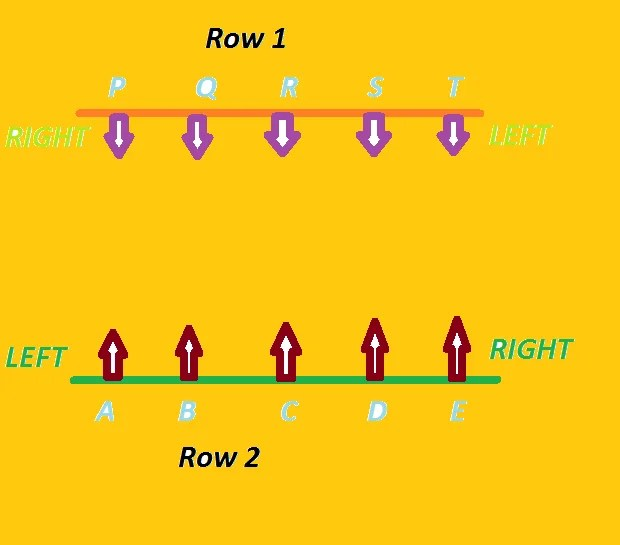How To Solve Row Seating Arrangement Problems – Tips And Tricks

Row Seating arrangement problems are always asked in the puzzles section of bank and insurance officers examinations. These questions could easily give you full marks in matter of 4-5 minutes. You just need to know basic rules to solve it and keep a cool and fresh mind during solving these problems. Puzzles questions are basically plays the important role in securing good marks in the reasoning. Candidates who have good grip over puzzles are mostly make it to the successful candidates list. Most important part of puzzle questions are reading the question very carefully. So Let’s continue with the rule and fast solving method of row seating arrangement problems.

Rules for Row Seating Arrangement Problems :

Basically there are two types of row seating arrangement problems are asked :

1. Single Row Seating Arrangement
2. Double Row Seating Arrangement

Rules for Single Single Row Seating Arrangement :

Persons Seating in the same row and all facing the same direction :Here A , B , C , D , E and F are sitting in the same and facing the same direction. A is at the extreme left of the row and F is at the extreme right of the row. C is immediate left to D and immediate right to B. D is 2nd right to B and 2nd left to F.

Rules for Double Row Seating Arrangement :

Persons Seating in Two Rows Facing Each Other ( Opposite Direction ) :In Row 1 , P is at the extreme right and T is at the extreme left of the row. In Row 2 , A is at the extreme left and E is at the extreme right of the row. P and A are facing each other and sitting opposite to each other. A and T are diagonally opposite to each other. Similarly Q and D are diagonally opposite to each other.

Let’s solve some problems now :

Example 1 :  Nine cricket fans are watching a match in a stadium. Seated in one row, they are J, K, L, M, N, O, P, Q and R. L is at the immediate right of M and at third place at the right of N. K is at one end of the row. Q is seated adjacent to both O and P. O is at the third place at the left of K. J is right next to left of O.

Which of the following statements is true ?

1. N is two seats away from J
2. M is at one extreme end
3. R and P are neighbours
4. There is one person between L and O

Solution 1 : Let’s draw the diagram based on the data provided and using rule of single row seating.

Therefore by using single row seating rule, we will get

Since L is at the immediate right of M but at the third right of N. Therefore , N __ M L is the first sequence.

Since K is at one end of the row But O is at the third left of K. Therefore __ __ __ __ __ O __ __ K is the second sequence.

Since Q is seated adjacent to O and P and J is next to left of O. Therefore N __ M L J O Q P K is the third sequence. Now we are only left with one person and one vacant place. Therefore the final sequence is

N R M L J O Q P KTherefore, answer to the given question is 3. There is one person between L and O that is J. So 3. is true.

Example 2 : Six persons A, B, C, D, E and F are sitting in two rows, three in each. E is not at the end of any row. D is second to the left of F. C, the neighbour of E, is sitting diagonally opposite to D. B is the neighbour of F.

Which of the following are sitting diagonally opposite to each other ?

1. F and C
2. D and A
3. A and C
4. A and F
5. A and B

Solution 2 : Now using the rule of two rows seating arrangement and given data, we get

Since Row 1 and 2 contains 3 persons each and E is not at the end of any row. It means E is at the middle of either of the rows . Therefore, the possibilities are

__ E __

__ E __

D is sitting left of F, Therefore Possibilities are

F  E  D                       __ E __

__ E __                       D  E  F

Now we know C is the neighbour of E, and sitting diagonally opposite to D. Therefore , the possibilities are

F  __ D                          __ E C

C  E  __                          D __ F

Since B is the neighbour of F, Therefore final diagram will be like

F B D                           A E C

C E A                           D B FSo therefore, answer to the question is 4. A and F are sitting diagonally opposite to each other.

This site uses Akismet to reduce spam. Learn how your comment data is processed.